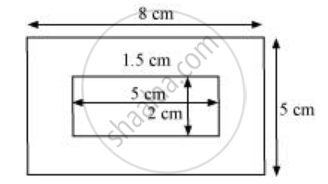Share

# A Picture is Painted on a Cardboard 8 Cm Long and 5 Cm Wide Such that There is a Margin of 1.5 Cm Along Each of Its Sides. Find the Total Area of the Margin. - CBSE Class 7 - Mathematics

ConceptConcept of Application of Conversion

#### Question

A picture is painted on a cardboard 8 cm long and 5 cm wide such that there is a margin of 1.5 cm along each of its sides. Find the total area of the margin.

#### SolutionLength (l) of cardboard = 8 cm

Breadth (b) of cardboard = 5 cm

Area of cardboard including margin = l × b = 8 × 5 = 40 cm2

From the figure, it can be observed that the new length and breadth of the cardboard, when margin is not included, are 5 cm and 2 cm respectively.

Area of the cardboard not including the margin = 5 × 2 = 10 cm2

Area of the margin = Area of cardboard including the margin − Area of cardboard not

including the margin

= 40 − 10 = 30 cm2

Is there an error in this question or solution?

#### APPEARS IN

NCERT Solution for Mathematics for Class 7 (2018 to Current)
Chapter 11: Perimeter and Area
Ex. 11.40 | Q: 3 | Page no. 226
Solution A Picture is Painted on a Cardboard 8 Cm Long and 5 Cm Wide Such that There is a Margin of 1.5 Cm Along Each of Its Sides. Find the Total Area of the Margin. Concept: Concept of Application of Conversion.
S